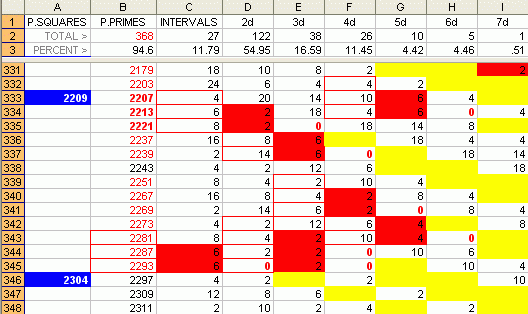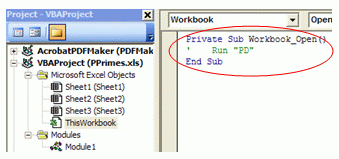dedicated to explicating prime numbers
without imaginary numbershome

First Published March 2007

Attention Math Students:
This topic is not related to
solving prime polynomials.

See Also
The High Primality of PPPs

 Poly Primes: The Polynomiality of Proximate Primes What's a Poly Prime? A poly prime is one that shares a common, or finite, difference with its proximate primes. Most primes - more than 90% of primes under 300,000 - exhibit a polynomial relationship to their neighboring primes. That is, their relationship to their preceding and proceeding primes can be described by a polynomial expression. For example, the quadratic formula for 17, 19, 23, and 29 is n^2 - n + 17. Polynomial primes can be linear (simply a common interval), quadratic (a common difference in the difference between the intervals), cubic (a common difference of the third degree), and so on through higher degrees of polynomiality. More primes resolve into a common difference of the 2nd degree than any other - that is, the relationship is explicable with a quadratic equation. (You can use QTest to explore and discover quadratic sequences.) For example, in the illustration, in the range between the perfect squares 2209 and 2304, there are 13 primes. Of these, all but one show a polynomial relationship to their adjacent primes. 2281, 2287, and 2293 have the simplest relationship, meaning they have equal intervals. This is a linear, or first degree, polynomial. 2207, 2213, and 2221 are 2nd degree polynomials - that is, their relationship can be described by a quadratic equation. (Bolded poly primes are all quadratic.) 2221 also shares a cubic polynomial relationship with 2237 and 2239 - a common difference of the 3rd degree. 2251, 2267, and 2269 have a 4th degree relationship.Download the Excel spreadsheet illustrated here to investigate the polynomiality of proximate primes. On opening the spreadsheet, the macro code will automatically execute for about 15 minutes, calculating common differences to the 30th degree for the first 26,000 primes (up to 300,000) . Download PPrimes.zip (20KB) Michael M. Ross Notes: Macro security must be Low or Medium to run. If you want to save the file and prevent it from re-executing the next time you open it, delete or comment out the autorun command as illustrated. (Tools > Macro > Visual Basic Editor > VBAProject (PPrimes.xls) > Microsoft Excel Objects > ThisWorkbook.)